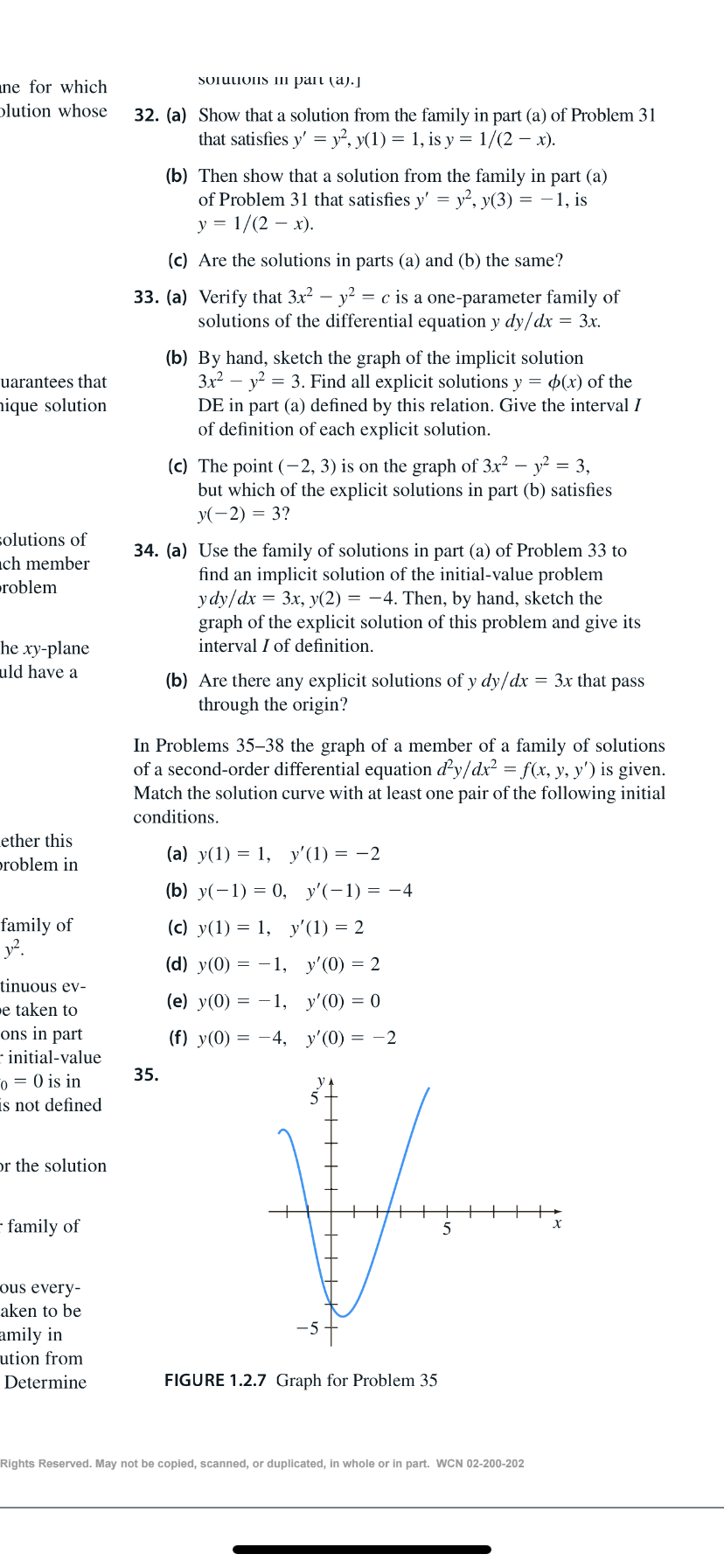# SOTUIONS Iipart (a).]ane for whichplution whose32. (a) Show that a solution from the family in part (a) of Problem 31that satisfies y' = y², y(1) = 1, is y = 1/(2 – x).(b) Then show that a solution from the family in part (a)of Problem 31 that satisfies y' = y², y(3) = –1, isy = 1/(2 – x).(c) Are the solutions in parts (a) and (b) the same?33. (a) Verify that 3x² – y² = c is a one-parameter family ofsolutions of the differential equation y dy/dx = 3x.(b) By hand, sketch the graph of the implicit solution3x – y = 3. Find all explicit solutions y = 4(x) of thein part (a) defined by this relation. Give the interval Iof definition of each explicit solution.uarantees thatnique solution(c) The point (-2, 3) is on the graph of 3x2 – y² = 3,but which of the explicit solutions in part (b) satisfiesy(-2) = 3?solutions of34. (a) Use the family of solutions in part (a) of Problem 33 toch memberroblemfind an implicit solution of the initial-value problemydy/dx = 3x, y(2) = -4. Then, by hand, sketch thegraph of the explicit solution of this problem and give itshe xy-planeuld have ainterval I of definition.= 3x that pass(b) Are there any explicit solutions of y dy/dxthrough the origin?In Problems 35–38 the graph of a member of a family of solutionsof a second-order differential equation dy/dx2 = f(x, y, y') is given.Match the solution curve with at least one pair of the following initialconditions.ether this(a) y(1) = 1, y'(1) = -2problem in(b) y(-1) = 0, y'(-1) = -4family ofy?.(c) y(1) = 1, y'(1) = 2(d) y(0) = -1, y'(0) = 2tinuous ev-(e) y(0) = -1, y'(0) = 0e taken toons in part· initial-valueo = 0 is inis not defined(f) y(0) = -4, y'(0) = -235.pr the solutionfamily ofхous every-aken to beamily inution fromFIGURE 1.2.7 Graph for Problem 35DetermineRights Reserved. May not be copied, scanned, or duplicated, in whole or in part. WCN 02-200-202

Question
20 viewshelp_outlineImage TranscriptioncloseSOTUIONS Ii part (a).] ane for which plution whose 32. (a) Show that a solution from the family in part (a) of Problem 31 that satisfies y' = y², y(1) = 1, is y = 1/(2 – x). (b) Then show that a solution from the family in part (a) of Problem 31 that satisfies y' = y², y(3) = –1, is y = 1/(2 – x). (c) Are the solutions in parts (a) and (b) the same? 33. (a) Verify that 3x² – y² = c is a one-parameter family of solutions of the differential equation y dy/dx = 3x. (b) By hand, sketch the graph of the implicit solution 3x – y = 3. Find all explicit solutions y = 4(x) of the in part (a) defined by this relation. Give the interval I of definition of each explicit solution. uarantees that nique solution (c) The point (-2, 3) is on the graph of 3x2 – y² = 3, but which of the explicit solutions in part (b) satisfies y(-2) = 3? solutions of 34. (a) Use the family of solutions in part (a) of Problem 33 to ch member roblem find an implicit solution of the initial-value problem ydy/dx = 3x, y(2) = -4. Then, by hand, sketch the graph of the explicit solution of this problem and give its he xy-plane uld have a interval I of definition. = 3x that pass (b) Are there any explicit solutions of y dy/dx through the origin? In Problems 35–38 the graph of a member of a family of solutions of a second-order differential equation dy/dx2 = f(x, y, y') is given. Match the solution curve with at least one pair of the following initial conditions. ether this (a) y(1) = 1, y'(1) = -2 problem in (b) y(-1) = 0, y'(-1) = -4 family of y?. (c) y(1) = 1, y'(1) = 2 (d) y(0) = -1, y'(0) = 2 tinuous ev- (e) y(0) = -1, y'(0) = 0 e taken to ons in part · initial-value o = 0 is in is not defined (f) y(0) = -4, y'(0) = -2 35. pr the solution family of х ous every- aken to be amily in ution from FIGURE 1.2.7 Graph for Problem 35 Determine Rights Reserved. May not be copied, scanned, or duplicated, in whole or in part. WCN 02-200-202 fullscreen
check_circle

star
star
star
star
star
1 Rating
Step 1

Observed from the given graph, that y (–1)...

### Want to see the full answer?

See Solution

#### Want to see this answer and more?

Solutions are written by subject experts who are available 24/7. Questions are typically answered within 1 hour.*

See Solution
*Response times may vary by subject and question.
Tagged in

### Differential Equations